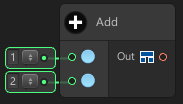# ArithmeticThe Arithmetic node is used to perform arithmetic operation.

There are 6 operator type to chose:

• Add is used to add a value to “Target A” with a value of “Target B”
• Subtract is used to substract a value to “Target A” with a value of “Target B”
• Divide is used to divide a value to “Target A” with a value of “Target B”
• Multiply is used to multiply a value to “Target A” with a value of “Target B”
• Modulo is used to modulo a value to “Target A” with a value of “Target B”
• Power is used to rank/power of a value to “Target A” with a value of “Target